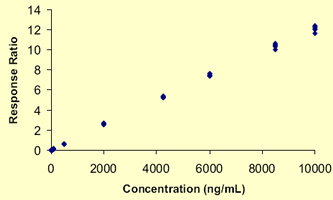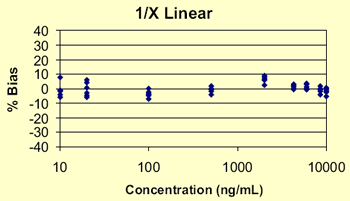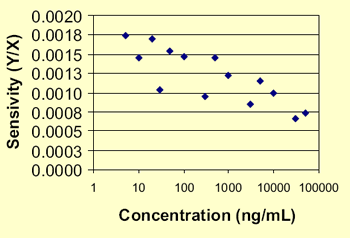# A Pre-validation Strategy For Evaluating Bioanalytical Methods #3186

J. Burrows1, N. Shearsby1, J. Chiu1, K. Watson1, A. Witkowski2
1BAS Analytics Ltd.: Kenilworth, United Kingdom
2BAS Analytics: West Lafayette, IN United States

#### Purpose

A generic approach is presented that provides concrete, statistical evidence for acceptable method performance prior to initiating a bioanalytical method validation.

#### Methods

A large number of replicate spiked matrix samples are analyzed in a single batch before a method validation is started. Careful selection of the contents of this batch enables one to evaluate factors such as the intra-assay precision, linearity, working range, maximum batch size, carryover and calibration design. Use of the linearity plot, sensitivity (y/x) versus log concentration (logx), rather than the usual response (y) versus concentration (x) graph, provides a critical way of visually evaluating the data.

#### Results

The utility of this pre-validation approach is illustrated using actual laboratory data. The process of interpreting the results and drawing conclusions about assay viability is demonstrated. The resulting conclusions provide sufficient background information to indicate if an assay is ready to enter the validation process.

#### General Design

• Initial R&D determines LC and sample prep conditions
• For LC/MS assays, evaluate potential matrix effects (ex. ion suppression, etc.) using neat versus extracted samples and infusion experiments before proceeding
• Prepare pre-validation batch as follows:
• 6 replicates of each calibration standard over the proposed range
(ex. 10 concentrations covering 1000-fold dynamic range)
• 6 additional replicates at 0.2xLLOQ, 0.5xLLOQ, and 2xULOQ
• 12 replicates of extracted blank matrix without ISTD
• at least one blank with ISTD added
• 6 different matrix blanks for specificity
• Inject samples in the following order: one replicate of each standard in increasing concentration, followed by 2 blanks without ISTD after highest standard, repeating this sequence 6 times. Include specificity blanks and blank(s) with ISTD where desired, along with any other samples of interest.
• Total batch size = ~ 100 samples

#### Data Analysis

Construct sensitivity plots (y/x versus x)

linear y = ax + b
quadratic y = ax2 + bx + c

if no signal observed in absence of analyte,
then intercept = 0 and

linear y/x = a
quadratic y/x = ax + b

Calculate accuracy, precision, and mean
%bias using various regression models

 Parameter Interpretation Precision As A Function Of Concentration Observe spread on sensitivity plot Estimate Of LLOQ Good linearity and precision < 10-15% Linearity Sensitivity plot flat for linear data and sloped line for quadratic data Appropriate Weighting Factor Simplest fit based on sensitivity plot, equal +/- distribution of % bias and smallest mean % bias Background Signal < 20% of LLOQ signal on sensitivity plot Maximum Batch Size And Autosampler Stability Look at assay performance as a function of injection order during batch Working Concentration Range Stay in linear portion if possible, with room above and below proposed dynamic range for daily variations in assay performance Carryover Check blanks following highest standards Specificity < 20% of LLOQ response in blank extracts

 Compound 1 - Simple Plot Compound 1 - Sensitivity PlotCompound 1 - % Bias Compound 1Regression Fit Mean % Bias Linear 75.67 1/X Linear 2.85 1/X2 Linear 2.81 Quadratic 4.80 1/X Quadratic 3.64 1/X2 Quadratic 2.78

 Compound 2 - Sensitivity Plot Compound 2Linear range = 1-250 ng/mL Starts to fall off at higher concentrations Poor precision and high background signal below 1 ng/mL

 Compound 3 - Sensitivity Plot Compound 3Only n=1 collected Precision obviously poor Not ready for prime time

#### CONCLUSIONS

Statistical evaluation of an assay’s characteristics before validation reduces the chance of attempting validation prematurely. This approach also provides an objective means for establishing the calibration range and (simplest) curve fitting scheme used. This simple pre-validation experiment ultimately leads to increased assay ruggedness.